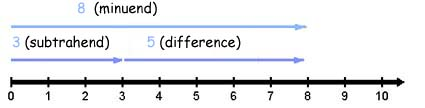Subtract Whole Numbers

Subtract Whole Numbers

Subtraction is the process of finding the difference between two numbers.

By counting backward, from 8 to 3, we see that the difference between

\$8 and \$3 is \$5.

\$8 - \$3 = \$5

(8,7,6,5,4,3)

Minuend - Subtrahend = Difference

Note from the number line that addition and subtraction are related. The fact that the sum of the subtrahend and difference equals the minuend can be used to check subtraction. Addition is the inverse operation of subtraction.The phrases below are used to indicate the operation of subtraction.

 Phrase Example Number Sentence minus 8 minus 5 8 - 5 less 10 less 3 10 - 3 less than 4 less than 7 7 - 4 the difference between the difference between 8 and 6 8 - 6 decreased by 5 decreased by 1 5 - 1

Subtract Large Numbers

• Arrange the numbers vertically
• Keep the digits that have the same place value in the same column
• Subtract the digits in each column, from right to left.

Practice

1. Subtract 6,483 - 3,261 and check.

2. Subtract 15,287 - 7,162 and check.

3. Subtract 17,504 - 9,202 and check.